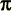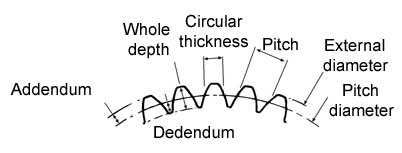Spur gears
A cylindrical spur gear is easily compared to a cylindrical friction wheel. As the latter, the former allows the transmission of a rotational movement between two parallel shafts, the ratio of angular velocities is determined by the ratio of the diameters of the wheels..However, whereas in the case of friction wheels, which are incapable of transmitting large forces and which, forcibly lead to a certain slippage, in the case of gears, the liason, obtained by obstacles, makes for a true transfer of power and can transmit considerable tork values.

The elements making up a toothed wheel which correspond to a friction wheel are called the pitch elements: pitch circle, cylindrical pitch, pitch circle diameter (PCD). These elements are very difficult to measure on a gear.

The coupling of two wheels working together is known as a gear. When the two wheels are of different diameters, the smaller of the two is known as a pinion, the larger - the gear.

The drive wheel governs the movement of the driven wheel.

A rack is considered to be a wheel of infinite diameter (it's pitch surface is a plane).

The cylindrical pitch of a gear is an imaginary cylinder whose surface form is found by meshing gears so that the surfaces actually touch each other (in other words - it is as if you are using two friction wheels of the same diameter). A straight section through this cylinder will give the pitch circle, which has a diameter d(PCD).

The external diameter is the diameter measured from the highest point on the teeth.

The base circle diameter is the diameter when measured from the root of the teeth.

The face is the portion of the surface of a tooth between the head and root.

The Circular Tooth Thickness is the length of the arc between the two faces of a tooth. Taken from the PCD.

The profile is a section through the face of the tooth. The form of this profile is known as an INVOLUTE CURVE.The pitch is the length of the arc, measured on the pitch circle, between similar points on two consecutive teeth. It is equal to the product ofand the module.

The module is used as a basis for all calculations of tooth dimension. This parameter may be found easily by a mathematical formula..

In order for two wheels to mesh they must have the same module (the same size of tooth). Two gears of different modules will be incompatible.

The dimensions of a spur gear are defined by the number of teeth and it's module. All other dimensions are linked to these two by The following formulas:STANDARDTOOTH Sym-bols Formulas Unit Module m pmm Pitch p m xmm PCD d Z x m mm number of teeth Z dm External diameter D (Z + 2) x m mm Addendum ha m mm Dedendum for  mod. 0,25 to 1,25 hf 1,40 x m mm Dedendum for 1,5 to 8 hf 1,25 x m mm Whole depth formod 0,25 ?nbsp;1,25 h 2,40 x m mm Whole depth formod 1,5 ?nbsp;8 h 2,25 x m mm Circular thickness e2 m mm

Consult tooth size for gears of module 0,20 to 6.

 Centre to centre a dA + dB2 The centre to centre distance tolerance is 0/+0.05 Speed ratios W1 = Z2W2 = Z1 W1 = Input speedW2 = Output speedZ1 = Number of teeth on drive wheelZ2 = Number of teeth on driven wheel

To assure continuous meshing, One couple of teeth must mesh before the previous pair seperates. In the case where there are a small number of teeth on a gear, a correction will be necessary to avoid interference between consecutive pairs of teeth.

Correction of teeth for gears of 8 to 17 teeth..
A tooth correction may be carried out on the gear to avoid interference which may occur at the base of the teeth. ATTENTION. This procedure will modify the PCD of the wheel, thus the centre to centre distance.

 Corrected teeth Symbols Formulas Unit Corrected PCD dc m (Z + 1) mm Corrected external diameter Dc m (Z + 3) mm

• Possibility of axial displacement,
• No axial forces,
• Reduction ratios of 2:1 to 40:1
• The simplest of gear systems
• Gears of the same module are interchangeable.How is the module calculated? The pitch circumference is : Pitch circumference =x PCD Also Pitch circumference = Number of teeth x Pitch Therefore : Number of teeth Z x Pitch (P) =x PCD Therefore :Where P = Pitch, d = PCD and Z = Number of teeth By definition, the resultant is known as the Module (m). It can be shown that :Therefore the basic formuli for gears may be found d = m Z p =m

From a geometric point of view, the module can be found on a tooth. It is the distance, in mm, between the Pitch arc and the External arc. For example, for module =2, there will be 2mm between the Pitch and External circles. Returning to the diameters, to find the external diameter it is necessary to add 2 x module to the PCD.

 Therefore one finds : d(ext) = m Z + 2m d(ext) = m (Z+2)

What is the shape of an involute curve?

This shape is defined by the trajectory of a point situated on a spur which will roll, without slipping, over a circle. This form has many advantages..

• There is a large tolerance in the distances between the gears before the operation of the system is affected.
• One single tool per module, allows for the machining of all sizes of wheel, however many teeth they have.
• The wear on the mating surfaces of the gears is much more evenly spread than on other profiles.
• Vibration levels are lower than other profiles.